# Excavation

Excavation for the base of the cottage has dimensions 4.5 m x 3.24 m x 60 cm. The excavated soil will increase its volume by one-quarter. Calculate the volume of excavated soil.

V =  10.935 m3

### Step-by-step explanation: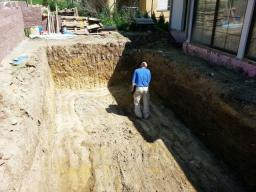Did you find an error or inaccuracy? Feel free to write us. Thank you!Tips to related online calculators
Need help to calculate sum, simplify or multiply fractions? Try our fraction calculator.
Do you want to convert length units?
Do you know the volume and unit volume, and want to convert volume units?

## Related math problems and questions:

• The excavation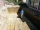The excavation for the city sewer was 38 m long, 2.2 m wide, and 3 m deep. a) How many cubic meters of soil were dredged b) How many journeys had to be made by one car when removing the soil if they loaded an average of 4.5 m of cubic soil on each journey
• Cuboid wallsThe block's base is a rectangle whose sides have lengths in the ratio of 13: 7. Find the volume of the block in liters if the longer side of the base measures 65 cm, and the height of the block is 1.2 m
• The cuboidThe cuboid is full to the brim with water. The external dimensions are 95 cm, 120 cm, and 60 cm. The thickness of all walls and the bottom is 5 cm. How many liters of water fit into the cuboid?
• Concrete boxThe concrete box with walls thick 7 cm has the following external dimensions: length 1.8 m, width 44 cm and height 46 cm. How many liters of soil can fit if I fill it to the brim?
• WaterInto a full cylindrical tank high 3 m with a base radius of 2.5 m, we insert cuboid with dimensions 1.7 m, 1.3 m, 1.9 m. How many liters of water will overflow out?
• The trench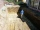Calculate how many cubic meters of soil needs to be removed from the excavation in the shape of an isosceles trapezoid, the top width is 3 meters, the lower width is 1.8 m, the depth of the excavation is 1 m, and the length is 20 m.
• Base of houseCalculate the volume of the bases of a square house, if the base depth is 1.2 m, the width is 40 cm and their outer circumference is 40.7 m.
• TetrapackHow high should be the milk box in the shape of a prism with base dimensions 8 cm and 8.8 cm if its volume is 1 liter?
• CardboardHow many m2 of cardboard are needed to make the cuboid with dimensions 40 cm 60 cm and 20 cm?
• Four prismsQuestion No. 1: The prism has the dimensions a = 2.5 cm, b = 100 mm, c = 12 cm. What is its volume? a) 3000 cm2 b) 300 cm2 c) 3000 cm3 d) 300 cm3 Question No.2: The prism base is a rhombus with a side length of 30 cm and a height of 27 cm. The height of t
• Trunk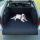Calculate length of the biggest fishing rod can be inserted into the trunk of a car with dimensions 165 x 99 × 85 cm?
• FlowerpotThe block-shaped flowerpot has external dimensions: length 1.25 m, width 10 cm, and height 11 cm. The thickness of the boards from which it is made is 0.8 cm. How many liters of soil is needed to fill it 1 cm below the top edge? What surface do we have to
• The wall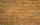We have to build a cuboid wall with dimensions base 30 cm and 45 cm and height 3.25 meters. Calculate how many we need bricks if we spend 400 pieces of bricks to 1 m3 of wall?
• Concrete tank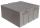How many m3 of concrete is in the tank shape of a cuboid with dimensions of 2000 cm and 5.6 meters and 70 dm if the concrete takes up 3/7 of the tank?
• Workers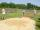Workers digging a jump pit in the schoolyard. The pit has a cuboid shape with a length 12 m, a width of 20 dm, and a depth of 36 cm. They excavate 0.4 cubic meters of soil an hour. How much time (hours and minutes) is need to excavate this pit?
• RainfallA rectangular garden of 25m in length and width 20m in width fall 4mm of water. Express by a fraction in basic form what part of the 60-hectolitre tank we would fill with this water.
• Mr. Gardener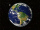Mr. Gardener wants to make wood for the balcony. Boxes. Each will have the shape of a perpendicular prism with a square base, the height is limited to 60 cm. Each container will be filled with soil by pouring the whole bag of substrate sold in a package w